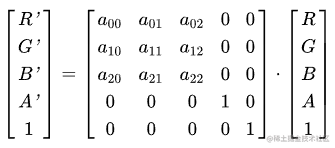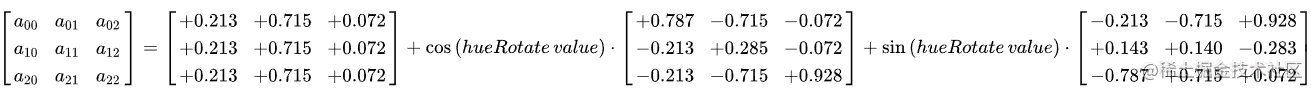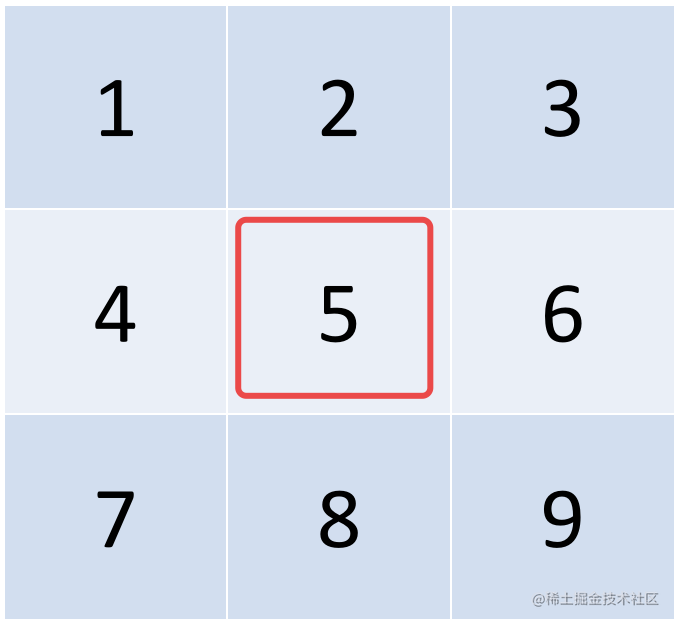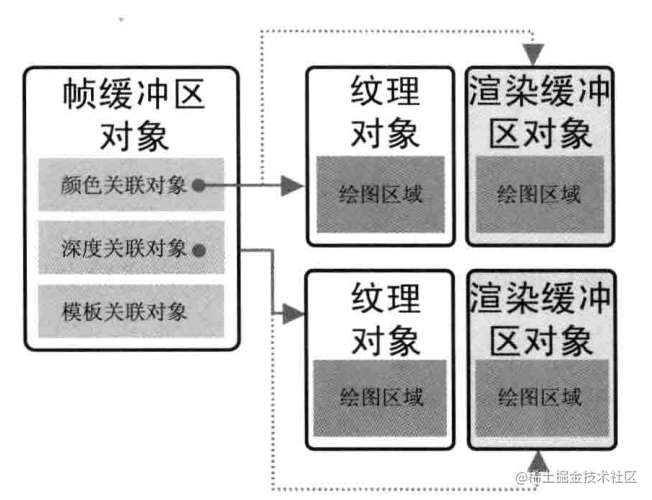# 前言

1. 灰度化（就是把彩色图像变成黑白）
2. 锐化
3. 模糊
4. 调色（色相、饱和度、对比度、色温等）
5. 其他LUT效果等（简单的说就是各类P图软件中一些常见的滤镜，比如 “夏日恋歌”，“赛博朋克”之类的滤镜）

# Let do it

## 黑白

### 原理

1. 让其中的两个通道的颜色变成另一个通道的颜色值，比如将 RGB中的 GB都等于R的值，或者让RB变成G的值。
2. 取RGB的平均值

``````precision mediump float;
varying vec2 v_texCoord;
uniform sampler2D u_texture;
void main () {
vec4 color = texture2D(u_texture, v_texCoord);
gl_FragColor = vec4(vec3(dot(color.rgb, vec3(0.333, 0.333, 0.333))), color.a);
}

`dot(color.rgb, vec3(0.333, 0.333, 0.333))` 这句话表示的就是对图像的RGB值求平均的意思。`dot`表示向量的点乘。他等价于： `color.r * 0.333 + color.g * 0.333 + color.b * 0.333`; 而 0.333约等于 `1 / 3`

1. 绘制图像到画布上
2. 通过getImageData获取画布的像素值
3. 遍历所有像素进行处理
4. 通过putImageData将处理好的数据绘制到画布上

``````  const imageData = ctx.getImageData(0, 0, width, height);
for (let y = 0; y < height; y++) {
for (let x = 0; x < width; x++) {
let r = imageData.data[(y * width + x) * 4];
let g = imageData.data[(y * width + x) * 4 + 1];
let b = imageData.data[(y * width + x) * 4 + 2];
let avg = (r + g + b) / 3.0;
imageData.data[(y * width + x) * 4] = avg;
imageData.data[(y * width + x) * 4 + 1] = avg;
imageData.data[(y * width + x) * 4 + 2] = avg;
}
}

ctx.putImageData(imageData, 0, 0);

## 调色

For type="hueRotate", values is a single one real number value (degrees). A hueRotate operation is equivalent to the following matrix operation:where the terms a00, a01, etc. are calculated as follows:`a00 = 0.213 + cos(hueRotate) * 0.787 + sin(hueRotate) * (-0.213)` 依次类推

`R' = a00 * R + a01 * G + a02 * B + 0 * A + 0 * 1`;
`G' = a10 * R + a11 * G + a12 * B + 0 * A + 0 * 1`;
`B' = a20 * R + a21 * G + a22 * B + 0 * A + 0 * 1`;
`A' = 0 * R + 0 * G + 0 * B + 1 * A + 0 * 1`;

``````precision mediump float;
varying vec2 v_texCoord;
uniform sampler2D u_texture;
uniform mat4 hueMat;
void main () {
vec4 color = texture2D(u_texture, v_texCoord);
gl_FragColor = hueMat * color;
}

（在WebGL概述——原理篇 中我讲述了如何向GPU中传递我们的参数）

``````export function createHueRotateMatrix(value) {
let sin = Math.sin((value * Math.PI) / 180);
let cos = Math.cos((value * Math.PI) / 180);
return new Float32Array([
0.213 + cos * 0.787 - sin * 0.213, 0.213 - cos * 0.213 + sin * 0.143, 0.213 - cos * 0.213 - sin * 0.787,0.0,
0.715 - cos * 0.715 - sin * 0.715, 0.715 + cos * 0.285 + sin * 0.14, 0.715 - cos * 0.715 + sin * 0.715, 0.0,
0.072 - cos * 0.072 + sin * 0.928, 0.072 - cos * 0.072 - sin * 0.283, 0.072 + cos * 0.928 + sin * 0.072, 0.0,
0.0, 0.0, 0.0, 1.0,
]);
}

``````const hueMat = createHueRotateMatrix(200);
for (let y = 0; y < height; y++) {
for (let x = 0; x < width; x++) {
let r = imageData.data[(y * width + x) * 4];
let g = imageData.data[(y * width + x) * 4 + 1];
let b = imageData.data[(y * width + x) * 4 + 2];
imageData.data[(y * width + x) * 4] = hueMat * r + hueMat * g + hueMat * b;
imageData.data[(y * width + x) * 4 + 1] = hueMat * r + hueMat * g + hueMat * b;
imageData.data[(y * width + x) * 4 + 2] = hueMat * r + hueMat * g + hueMat * b;
}
}

## 模糊效果

### 原理``````precision mediump float;
varying vec2 v_texCoord;
uniform sampler2D u_texture;
uniform vec2 u_resolution;
void main () {
vec2 one_pixel = 1. / u_resolution;
vec4 color = texture2D(u_texture, v_texCoord);
vec4 l = texture2D(u_texture, v_texCoord + vec2(-1.0, 0.0) * one_pixel);
vec4 r = texture2D(u_texture, v_texCoord + vec2(1.0, 0.0) * one_pixel);
vec4 t = texture2D(u_texture, v_texCoord + vec2(0.0, -1.0) * one_pixel);
vec4 b = texture2D(u_texture, v_texCoord + vec2(0.0, 1.0) * one_pixel);
gl_FragColor = vec4((l + r + t + b + color).rgb / 5., color.a);
}## Framebuffer

`Framebuffer`中文名为“帧缓冲”对象。在默认情况下，WebGL在颜色缓冲区中进行绘图。总之，绘制的结果图像是存储在颜色缓冲区中的。帧缓冲区对象可以用来代替颜色缓冲区或者深度缓冲区（这里我们不考虑深度缓冲区）。在帧缓冲区中绘制的过程又称为离屏绘制。``````
export function createFramebufferTexture(gl, number, width, height) {
let framebuffers = [];
let textures = [];
for (let i = 0; i < number; i++) {
let framebuffer = gl.createFramebuffer();
gl.bindFramebuffer(gl.FRAMEBUFFER, framebuffer);
// 这里的texture就是颜色关联对象，它替代了颜色缓冲区
let texture = createTexture(gl);
gl.texImage2D(gl.TEXTURE_2D, 0, gl.RGBA,width,height,0,gl.RGBA,gl.UNSIGNED_BYTE,null);
// 将texture与framebuffer关联起来
gl.framebufferTexture2D(gl.FRAMEBUFFER,gl.COLOR_ATTACHMENT0,gl.TEXTURE_2D,texture,0);
texture && textures.push(texture);
framebuffer && framebuffers.push(framebuffer);
}
gl.bindFramebuffer(gl.FRAMEBUFFER, null);
return [framebuffers, textures];
}

``````function drawByShader() {
// 设置原始纹理为第一次的输入
gl.bindTexture(gl.TEXTURE_2D, texture);
for (let i = 0; i < 10; i++) {
// 进入到其中一个帧缓冲区
gl.bindFramebuffer(gl.FRAMEBUFFER, framebuffers[i % 2]);
// 绘制到当前帧缓冲区中的纹理对象上
gl.drawArrays(gl.TRIANGLES, 0, 6);
// 绑定当前缓冲区的纹理对象作为下一次处理的输入
gl.bindTexture(gl.TEXTURE_2D, textures[i % 2]);
}
// 设置帧缓冲区为NULL时，则会绘制到颜色缓冲区中（即屏幕上）
gl.bindFramebuffer(gl.FRAMEBUFFER, null);
gl.drawArrays(gl.TRIANGLES, 0, 6);
}

``````
function drawImage(img) {
ctx.drawImage(img, 0, 0);
const width = canvas2.width;
const height = canvas2.height;

const imageData = ctx.getImageData(0, 0, width, height);
// 做20次的重复处理
for (let i = 0; i < 20; i++) {
processImageData(imageData, (x, y, texture2D) => {
const color = texture2D(x, y);
const l = texture2D(x - 1, y);
const r = texture2D(x + 1, y);
const t = texture2D(x, y - 1);
const b = texture2D(x, y + 1);
const sum = [color, l, r, t, b].reduce((prev, cur) => {
return [
prev + cur,
prev + cur,
prev + cur,
prev + cur,
]
}, [0, 0, 0, 0]);
return sum.map(item => item /= 5);
});
}
ctx.putImageData(imageData, 0, 0);
}

function processImageData(
imageData,
processFunc = (x, y, texture2D) => {
return texture2D(x, y);
}
) {
const width = imageData.width;
const height = imageData.height;
const texture2D = (x, y) => {
if (x < 0) {
x = 0;
}
if (x >= width) {
x = width - 1;
}
if (y < 0) {
y = 0;
}
if (y >= height) {
y = height - 1;
}
let r = imageData.data[(y * width + x) * 4];
let g = imageData.data[(y * width + x) * 4 + 1];
let b = imageData.data[(y * width + x) * 4 + 2];
let a = imageData.data[(y * width + x) * 4 + 3];
return [r, g, b, a];
}

for (let y = 0; y < height; y++) {
for (let x = 0; x < width; x++) {
[
imageData.data[(y * width + x) * 4],
imageData.data[(y * width + x) * 4 + 1],
imageData.data[(y * width + x) * 4 + 2],
imageData.data[(y * width + x) * 4 + 3]
] = processFunc(x, y, texture2D);
}
}
}

Bloom效果是一种游戏中常见的效果，它让画面中较亮的区域像是“扩散”到了周围的区域中，造成一种朦胧的效果。# 总结

1. 黑白：r,g,b值相等时图片就会呈现黑白的表示形式。
2. 色相：我们通过查阅W3C标准，找到相应的矩阵表示形式，我们通过矩阵乘法完成了色相旋转。